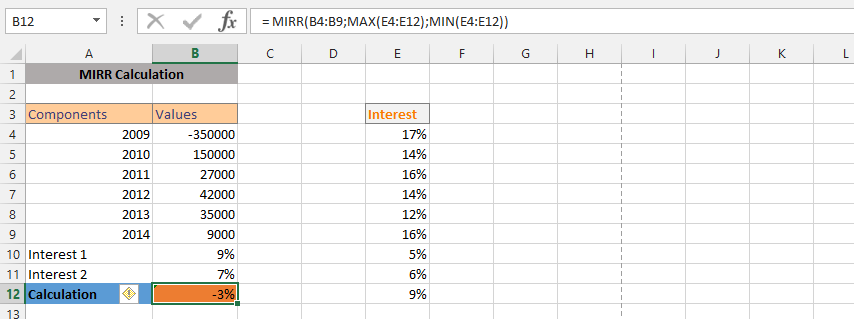#### How to use the Excel MIRR function?

The term MIRR (Modified Internal Rate of Return) is commonly used in corporate financial or financial management to indicate the profitability of an investment, and is therefore commonly used in making business decisions when choosing between investments. The MIRR calculation uses a series / payment schedule (which includes the initial outflow along with net income / gains), when calculating the MIRR of interest capitalization, it is assumed that the Net Present Value of the investment is zero.

## The basics of the Mirr function

The main difference between MIRR and IRR (internal rate of return) is that the calculation of the MIRR takes into account the initial cost of the investment as well as the interest received on reinvestment of cash (compounding), while the IRR is calculated without compounding.

MIRR is an improved version of their IRR approach to capital budgeting decisions. Does not require the assumption that project cash flows are reinvested at an internal rate of return; it contains a rather discrete reinvestment rate in the model.

Decision rule: accept MIRR of projects larger than (project) threshold; in the case of mutually exclusive projects, a project with a higher MIRR should be selected.

Note: When calculating the MIRR, the cash flows should be at regular intervals but need not be the same for each interval.

## Syntax of the MIRR function in Excel

=MIRR( range, finance_rate, reinvestment_rate )

Parameters:

• range – is a range of cells that represent a series of cash flows (investment values ​​and net income) that occur at regular time periods. Must have at least one negative value (representing payment) and at least one positive value (representing income)
• finance_rate – This is the interest rate you pay on cash flow amounts.
• reinvestment_rate – This is the interest rate you get on the cash flow amounts when they are reinvested.

## Examples of the Mirr function

### Choice between two projects

Suppose you are a financial manager at some investment firm and you work for an engineering firm. Two of the company’s recent offers have been accepted.

The first is related to the construction of a new railway line, the second to the construction of a motorway. Both projects are to last 3 years. The applicable funding rate is 10% and the project’s cash flows (in dollars) are listed below: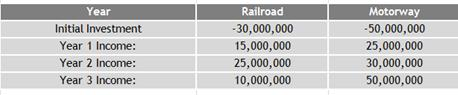Both projects had positive net present values.

Please recommend which project the company should accept. You believe the economy may slow down a bit in the next few years and a lower reinvestment rate should be considered. Your boss asked you to calculate the MIRR for both projects.

The MIRR function is used to calculate the MIRR for both projects as shown in the spreadsheet below. It uses a financial rate of 10% and a reinvestment rate of 8%.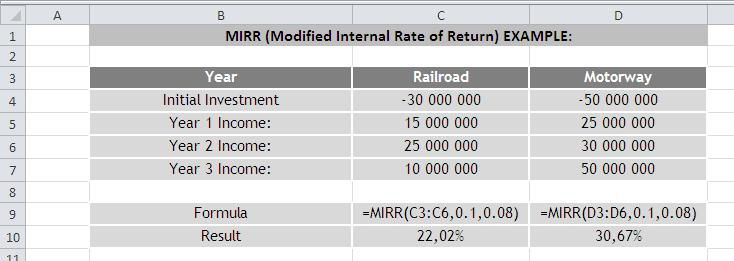The motorway project should be preferred based on the MIRR approach.

### Simple MIRR formula

The client is considering an investment and came to the broker to find out after five years how much the interest rate is worth. Traditionally, we just informed the client. But the client is persistent and determined to confirm the information himself. For this reason, we have set up MIRR so that he can see the details.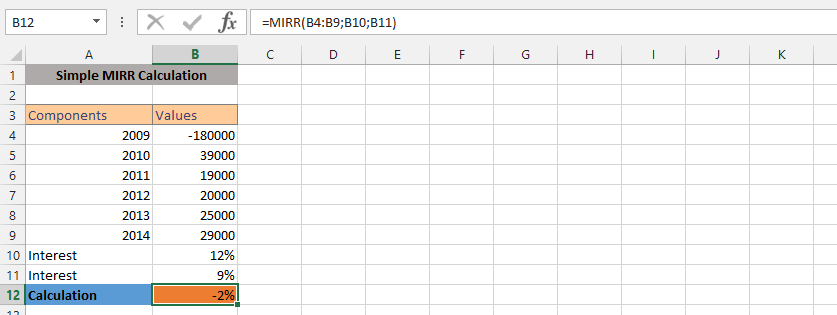### More detailed Mirr formula

The client wants to experiment with the calculations a bit and therefore it became necessary to use the detailed information to confirm the potential interest in the details.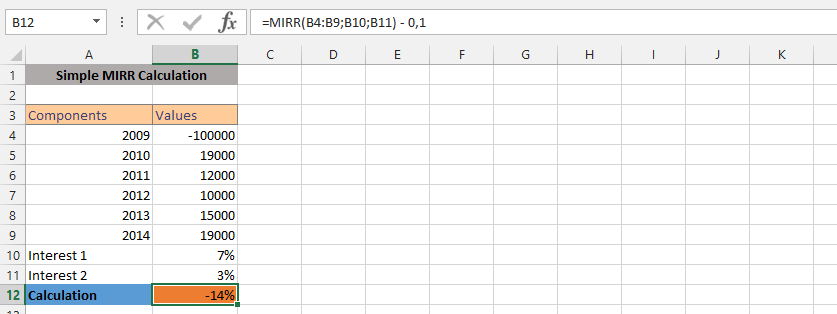### Flexibility in MIRR

With this information, the bank determines the amount of interest, if an interest rate has been specified. MIRR was the perfect and logical option to confirm how things would turn out knowing that the client already knows how much they have invested and how it has affected their current financial situation. They wonder how easily they would have made more money if the interest rate were fixed.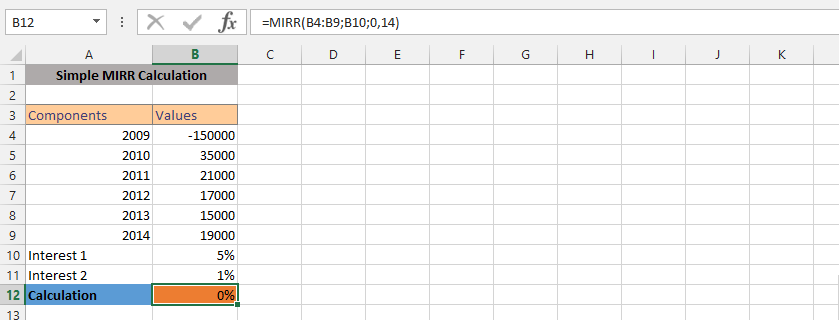### MIRR and Average

The company wants to evaluate a policy that also includes looking at the average performance of the entire investment. Therefore, we will use both the mean and MIRR functions for confirmation.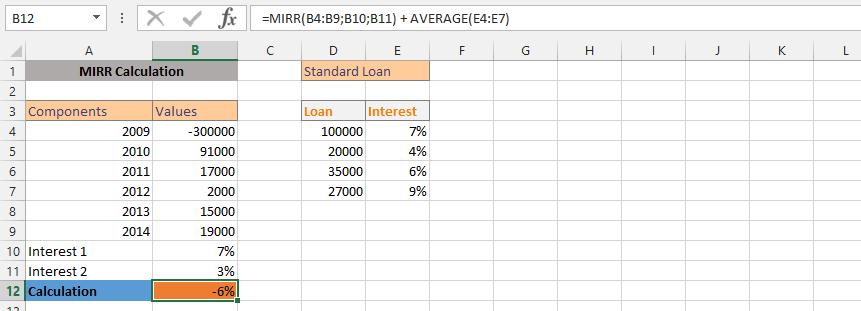### Average and MIRR

In this case, the situation is completely different. The client is stunned by the circumstances and wants to know what’s best for him. This time, we would like to know what the rate has been over the last year, which has given us productive data. Therefore, we will use the formula AVERAGE and MIRR in one formula.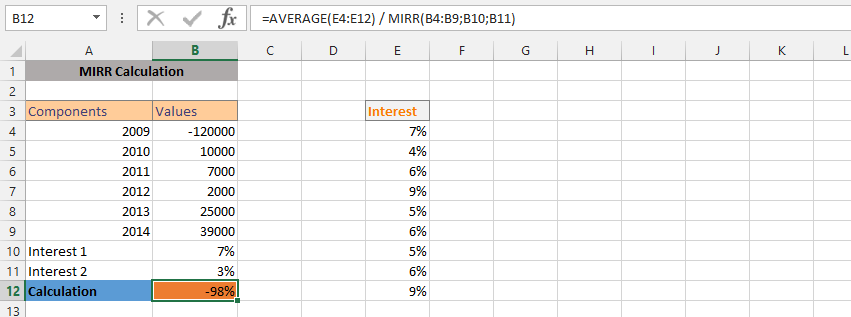### MIN and MIRR

The company has a long history of various rates and has been around for a long time. We have had different rates for years, and now we choose the minimum rate for the circumstances, so now we’re using both the Min and MIRR formula.### What is MIRR of 350,000?

We have a client who has 350,000 and is trying to figure out how much the money would be worth after fixing the interest rate. This allows you to confirm that we need to format the cell to currency after entering the formula.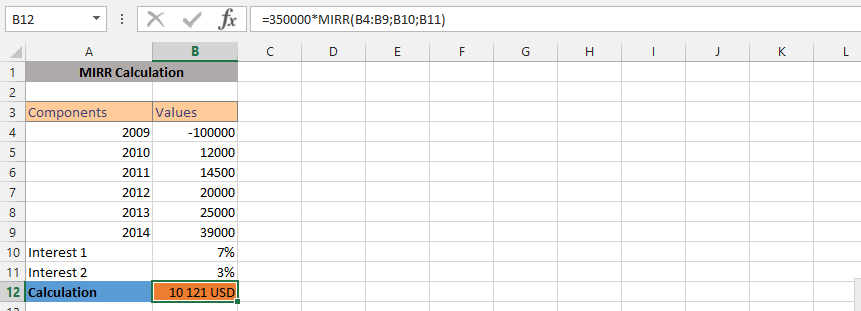### IF and MIRR

The client asks a question that involves us, confirming that we need to find a way to see how the show will work. The client asks for an interest on the investment and we would know that the client must have a certain amount in the investment account for this MIRR to be possible. Therefore, we use both IF and MIRR formulas to find the answer.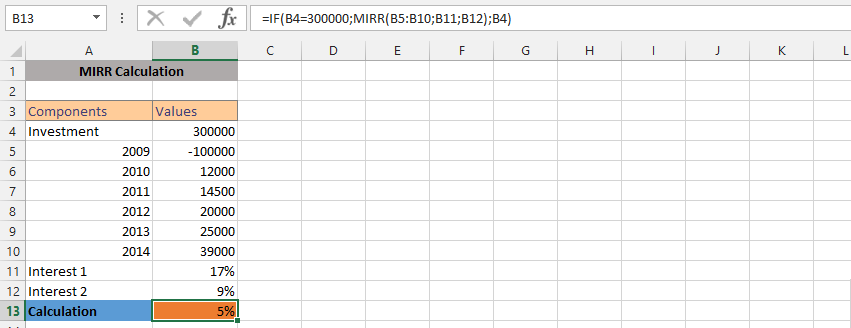### MAX and MIRR

The company evaluates how to use the information that is currently in business, and we prefer to use the MAX and MIRR formulas to find answers. All our interest rates have been fixed, but we rather use the MAX formula.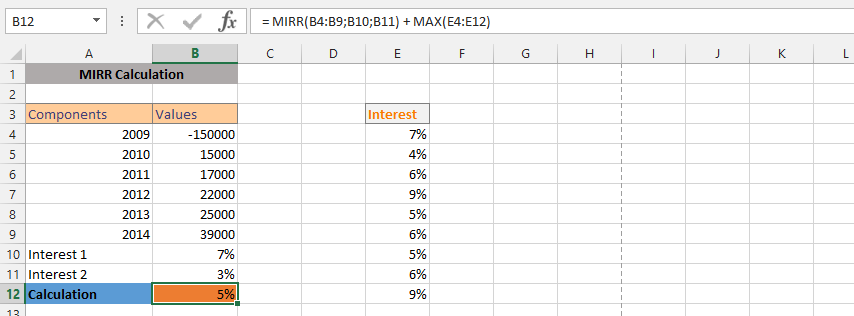### MIRR, MAX and MIN

In this example, the situation is completely different and we are not making an investment and interest rate that would work effectively to find a balance between customer and business. This is where MIRR, MAX and MIN work together to deliver a perfect result.